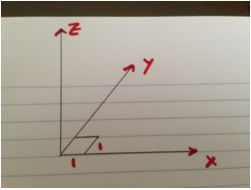# A simple sumThis calculation was inspired, a few years ago, by trying to find a simple way to explain the sum of the first$N$ natural numbers to my (then) twelve-year-old daughter, without the use of calculus. As many people know, the sum of the first$N$ natural numbers is found very easily, using the method that Gauss (apparently) re-discovered as a two-year old, i.e.,$S_N^1 = 1 + 2 + 3 + ... + N$

and$S_N^1=N+(N-1)+(N-2)+(N-3)+...+1$$2 (S^1_N) = N(N+1)$

i.e.,$S^l_N = \frac{N(N+1)}{2}$

Of course, this method cannot be used to sum up the series with squares or higher powers.

Let’s however, continue to use the convenient notation for the sum of squares$S_N^2 = 1^2+2^2+3^2+...+N^2$

There’s a useful recursion relation between$S_N^2$ and$S^2_{N-1}$$S_N^2= S_{N-1}^2+N^2$

Let’s imagine the following – say you have$1 \times 1$ (one-unit by one-unit) squares of fixed height cut out of paper. Suppose you arrange them first as below${\bf Layer 0}$there are$1^2$ pieces of paper here${\bf Layer 1}$there are$2^2$ pieces of paper here

And another layer,${\bf Layer 2}$there are$3^2$ pieces of paper here

Let’s place the pieces of paper so that$Layer 0$ is at the bottom,$Layer 1$ is next on top of it,$Layer 2$ is on top of that and so on.

Let’s compute the heights of the “skyscraper” that results!

The highest tower is the one on top of the square with vertices$(x=0, y=0), (x=1, y=0), (x=0,y=1)$ and$(x=1,y=1)$. It has height$N$ and the total number of pieces of paper in it are$N \times 1 = (N - 0) \times (1^2 - 0^2)$ square pieces of paper.

I’ve just written this in a suggestive way.

The next tower is the one just surrounding this one on two sides, there are actually three towers, of height$(N-1)$ and the total number of pieces of paper in it is$N \times 3 = (N-1) \times (2^2 - 1^2)$ square pieces of paper

Again, this is written in a suggestive way

The next tower is the one surrounding this last tower on two sides, there are five of them, of height$(N-2)$ and the total number of pieces of paper in it is$(N-2) \times 5 = (N-2) \times (3^2 - 2^2)$ square pieces of paper.

Yet again!

In general, the$k^{th}$ skyscraper has height$(N-k)$ and there are$((k+1)^2-k^2)$ of them, so the total number of square pieces of paper in it are$(N-k) \times ((k+1)^2 - k^2)$

Note, for later use, that the term$((k+1)^2-k^2)$ derives from the difference in the total number of pieces of$1 \times 1$ square paper that form a$k \times k$ square vs. a$(k+1) \times (k+1)$ square.

Adding this up for the remaining, we are left with the total number of square$1 \times 1$ pieces of paper, which is, indeed,$S_N^2 = 1^2+2^2+3^2 +4^2 ...+N^2$.

Writing this in summation notation$S_N^2 = \sum\limits_{k=0}^{N-1} (N-k) \times (2 k+1)$

which can be expanded into$S_N^2 = \sum\limits_{k=0}^{N-1} (2 N k + N - 2 k^2 - k)$

i.e.,$S_N^2 = 2 N \frac {N (N-1)}{2} + N^2 - 2 S_{N-1}^2 - \frac{N(N-1)}{2}$

Using our useful result from above$S_{N-1}^2 = S_N^2 - N^2$

We find$S_N^2 = \frac {N(N+1)(2 N+1)}{6}$

Aha – now we can generalize this!

We can continue this for the sum of the cubes of integers and so forth. Let’s start with$1 \times 1 \times 1$ cubes that are placed, starting at the origin. Again, using the notation$S_N^3 = 1^3+2^3+3^3+...+N^3$

Again, we note$S_N^3 = S_{N-1}^3 + N^3$

Continuing in the same vein, alas, we cannot layer the cubes on top of each other in three dimensions! Let’s assume there is indeed a fourth dimension and count the heights in this dimension – see, physicists and mathematicians are naturally led to higher dimensions! The number of cubical pieces used are found by counting the numbers in the expanding “perimeter” of cubes, just as in the two-dimensional example.$N \times 1 = (N-0) \times ( 1^3 - 0^3)$$(N-1) \times 8 = (N-1) \times (2^3 -1^3)$$(N -2) \times 19 = (N-2) \times (3^3 - 2^3)$$(N-3) \times 27 = (N-3) \times (4^3 - 3^3)$$(N-k) \times ( (k+1)^3 - k^3)$

So we are left with$S_N^3 = \sum\limits_{k=0}^{N-1} (N-k) \times (3 k^2+3 k+1)$

Which results in the usual formula (using the auxiliary relation$S_{N-1}^3=S_N^3-N^3$,$S_N^3= ((N(N+1))/2)^2$

In general, using this approach, and the auxillary relation$S_N^L= S_{N-1}^L+ N^L$

We find$S_N^L= \frac {1}{L+1} ( N^2 + L N^L + \frac{N+1}{L+1} [ C(L+1,1) L S_{N-1}^1$$+ C(L+1,2) (L-1) S_{N-1}^2 + C(L+1,3) (L-2) S_{N-1}^3$$+ ... + C(L+1,L-1) (L - [L-2]) S_{N-1}^{L-1} ] )$

where$C(n,m) = \frac{n!}{m! (n-m)!}$ is the combinatorics formula for the number of ways to select$m$ items out of$n$.

This formula, while original (as far as I can tell) in its derivation,  is consistent with Faulhaber’s formula from the 16th century.